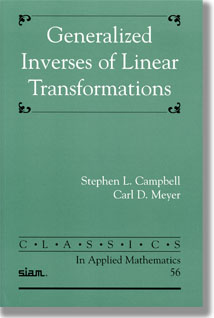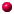# Generalized Inverses of Linear Transformations

### ISBN-10: 0898716713, ISBN-13: 978-0898716719 [QA188.C36 1991], 292 pages

This edition is number CL56 in the SIAM series CLASSICS in Applied Mathematics.
The original edition was published by Pitman Publishing Limited, London, 1979,
in the series Surveys and Reference Works in Mathematics, and was reprinted by
Dover Publications in 1991 (ISBN 0-486-66693-X).

Available from SIAM Books (at a discount) and from Amazon.com

##CONTENTS

• Preface to the Classics Edition
• Preface

1. The Moore-Penrose or generalized inverse
1. Basic definitions
2. Basic properties of the generalized inverse
3. Computation of the Moore-Penrose inverse
4. Generalized inverse of a product
5. Exercises

2. Least squares solutions
1. What kind of answer does the Moore-Penrose inverse produce?
2. Fitting a linear hypothesis
3. Estimating the unknown parameters
4. Goodness of fit
5. An application to curve fitting
6. Polynomial and more general fittings
7. Why use the Moore-Penrose inverse?

3. Sums, partitioned matrices, and the constrained generalized inverse
1. The generalized inverse of a sum
2. Modified matrices
3. Partitioned matrices
4. Block-triangular matrices
5. The fundamental matrix of constrained minimization
6. Constrained least squares and constrained generalized inverses
7. Exercises

4. Partial isometries and EP matrices
1. Introduction
2. Partial isometries
3. EP matrices
4. Exercises

5. The generalized inverse in electrical engineering
1. Introduction
2. n-port networks and impedance matrix
3. Parallel sums
4. Shorted matrices
5. Other uses of the generalized inverse
6. Exercises
7. References and further reading

6. (i,j,k)-Generalized inverses and linear estimation
1. Introduction
2. Definitions
3. (1)-inverses
4. Applications to the theory of linear estimation
5. Exercises

7. The Drazin inverse
1. Introduction
2. Definitions
3. Basic properties of the Drazin inverse
4. Spectral properties of the Drazin inverse
5. The Drazin inverse as a polynomial
6. The Drazin inverse as a limit
7. The Drazin inverse of a partitioned matrix
8. Other properties

8. Applications of the Drazin inverse to the theory of finite Markov Chains
1. Introduction and terminology
2. Introduction of the Drazin inverse into the theory of finite Markov chains
3. Regular chains
4. Ergodic chains
5. Calculation of the group inverse and the station distribution for an ergodic chain
6. Non-ergodic chains and absorbing chains
7. References and further reading

9. Applications of the Drazin inverse
1. Introduction
2. Applications of the Drazin inverse to linear systems of differential equations
3. Applications of the Drazin inverse to difference equations
4. The Leslie population growth model and backward population projection
5. Optimal control
6. Functions of a matrix
7. Weak Drazin inverses
8. Exercises

10. Continuity of the generalized inverse
1. Introduction
2. Matrix norms
3. Matrix norms and invertibility
4. Continuity of the Moore-Penrose generalized inverse
5. Matrix valued functions
6. Non-linear least squares problems: an example
7. Other inverses
8. Exercises
9. References and further reading

11. Linear programming
1. Introduction and basic theory
2. Pyle's reformulation
3. Exercises
4. References and further reading

12. Computational concerns
1. Introduction
2. Calculation of the Moore-Penrose inverse
3. Computation of the singular value decomposition
4. (1)-inverses
5. Computation of the Drazin inverse
6. Previous algorithms
7. Exercises

13. Bibliography and Index